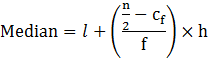Guru

# The Life insurance agent found the following data for the distribution of ages of 100 policy holders. Calculate the median age, if policies are given only to the persons whose age is 18 years onwards but less than the 60 years. Q.3

• 0

What is the best way for solving the problem of question no,3 of statistics chapter of exercise 14.3, how i solve this tough question in easy way give me the best and the simple way for solving this problem The Life insurance agent found the following data for the distribution of ages of 100 policy holders. Calculate the median age, if policies are given only to the persons whose age is 18 years onwards but less than the 60 years.

Share

1. This answer was edited.

Given data: n = 100 and n/2 = 50

Median class = 35-45

Then, l = 35, cf = 45, f = 33 & h = 5Median = 35+((50-45)/33) × 5

= 35 + (5/33)5

= 35.75

Therefore, the median age = 35.75 years.

• 0